A versatile cross-platform mind mapping tool.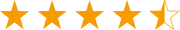Get from App StoreA versatile cross-platform mind mapping tool.Get from App Store

# Matrix Diagram for Commonly Used Matrix Diagrams

> Business Diagram Examples > Matrix Diagram for Commonly Used Matrix Diagrams
Matrix Diagram for SWOT, Ansoff matrix, BCG matrix, Authority matrix, position matrix and six sigma matrix - Easy to draw commonly used matrix diagrams.

### Matrix Diagram Software

Edraw Max is a vector-based Matrix Diagram software with diversified examples and templates for financial and sales reports, profit and loss statements, budgets, statistical analyses, accounting tasks, market projections and annual reports.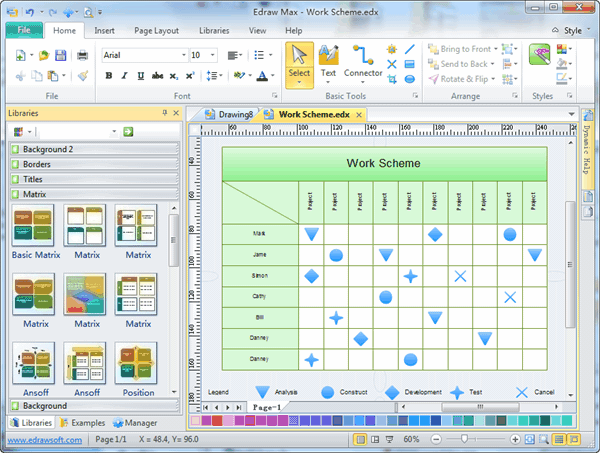### Matrix Diagram Template

This charting template helps you draw just about any imaginable chart or graph in considerable detail. You'll find all of the usual variations of the bar, pie, and line graph, including 3-D versions of each. In addition, you have access to various matrix diagram, grid and chart and graph additions. For example, you can add annotations to the charts and graphs. Because you can easily include data from multiple sources, this particular template is often more flexible than similar charting and graphing in a spreadsheet. The ability to add various diagrams into the chart or graph lets this template well surpass most spreadsheets.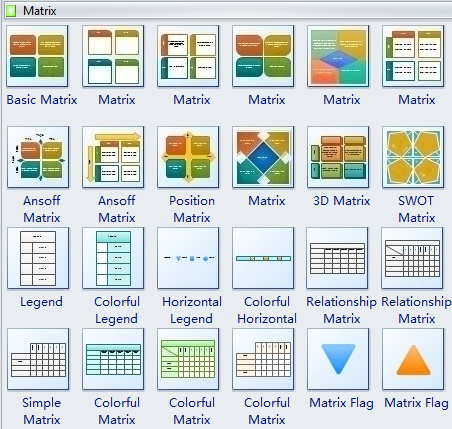### Basic Matrix Diagram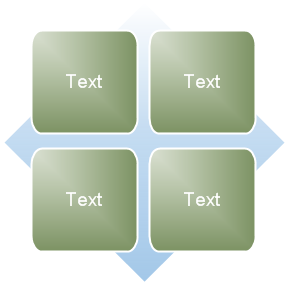It is used to show the relationship of components to a whole in quadrants. The first four lines of Level 1 text appear in the quadrants. Unused text does not show, but remains available if you switch layouts.

### Grid Matrix Diagram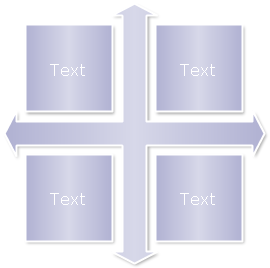This is used to show the placement of concepts along two axes. It emphasizes the individual components rather than the whole. The first four lines of Level 1 text appear in the quadrants. Unused text does not appear, but remains available if you switch layouts.

### Titled Matrix Diagram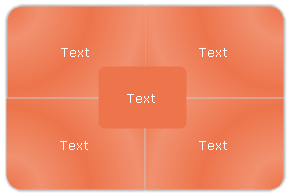People use it to illustrate the relationships of four quadrants to a whole. The first line of Level 1 text corresponds to the central shape, and the first four lines of Level 2 text appear in the quadrants. Unused text does not appear, but remains available if you switch layouts.

### Matrix Diagram Example

An example matrix diagram shows a simple project progress schedule.More Matrix Diagram Examples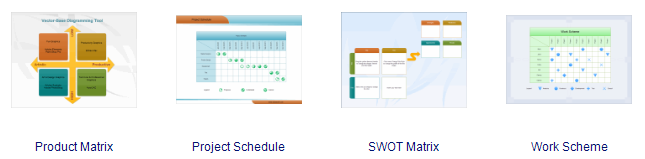SWOT Matrix Diagram

Ansoff Matrix Template

Relationship Matrix

Process Steps Diagram